## Bessel Function of the Second KindA Bessel function of the second kindis a solution to the Bessel Differential Equation which is singular at the origin. Bessel functions of the second kind are also called Neumann Functions or Weber Functions. The above plot showsfor, 2, ..., 5.

Let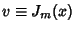be the first solution andbe the other one (since the Bessel Differential Equation is second-order, there are two Linearly Independent solutions). Then(1)(2)

Take(1) minus(2),(3)(4)

so, whereis a constant. Divide by,(5)(6)

Rearranging and usinggives(7)

where the Bessel function of the second kind is defined by(8), 1, 2, ...,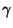is the Euler-Mascheroni Constant, and(9)

The function is given by(10)

Asymptotic equations are(11)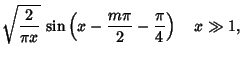(12)

where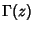is a Gamma Function.

See also Bessel Function of the First Kind, Bourget's Hypothesis, Hankel Function

References

Abramowitz, M. and Stegun, C. A. (Eds.). Bessel Functionsand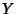.'' §9.1 in Handbook of Mathematical Functions with Formulas, Graphs, and Mathematical Tables, 9th printing. New York: Dover, pp. 358-364, 1972.

Arfken, G. Neumann Functions, Bessel Functions of the Second Kind,.'' §11.3 in Mathematical Methods for Physicists, 3rd ed. Orlando, FL: Academic Press, pp. 596-604, 1985.

Morse, P. M. and Feshbach, H. Methods of Theoretical Physics, Part I. New York: McGraw-Hill, pp. 625-627, 1953.

Spanier, J. and Oldham, K. B. The Neumann Function.'' Ch. 54 in An Atlas of Functions. Washington, DC: Hemisphere, pp. 533-542, 1987.